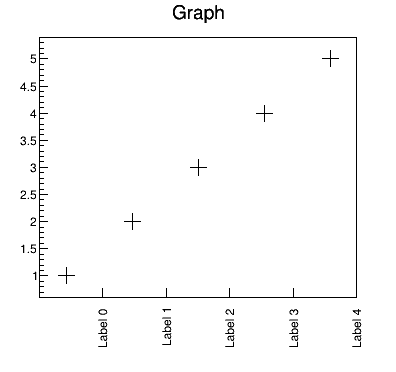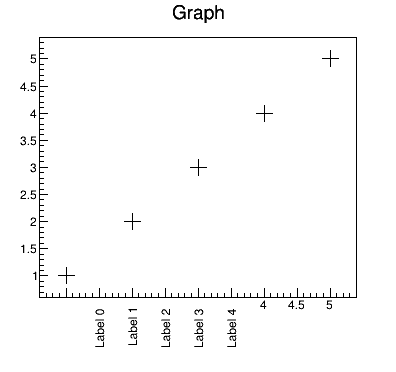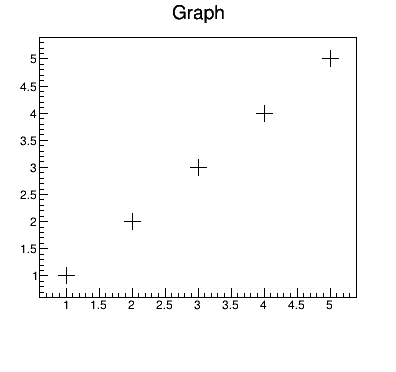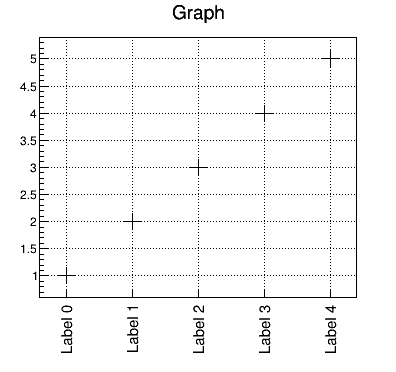# Aligning custom x-axis labels to bins in TGraph

Hello,

I am trying to set custom labels to my bins in TGraph. I figured out that I must use the `ChangeLabel` method on the axis. I also set `Ndivisions` so that the number of ticks correspond to the number of bins that I have. However, the ticks are not aligned with the bins.

Reproducible example:

``````import ROOT
import numpy as np

canvas = ROOT.TCanvas(f"test_canvas", "title", 400, 400)
canvas.SetBottomMargin(0.2)
canvas.Draw()
canvas.cd()

num_values = 5

g = ROOT.TGraph(num_values,
np.arange(num_values, dtype='float') + 1,
np.arange(num_values, dtype='float') + 1)
g.SetMarkerSize(2)
g.SetMarkerStyle(2)

xaxis = g.GetXaxis()
xaxis.SetNdivisions(-(num_values + 0))
xaxis.ChangeLabel(1, -1, -1, -1, -1, -1, " ")

labels=[f'Label {i}' for i in range(num_values)]

for i, label in enumerate(labels):
xaxis.ChangeLabel(i + 2, 90, -1, 32, -1, -1, label)

g.DrawClone('AP')
``````

Result:If I don’t use the Ndivisions, I get the following result instead:For completeness, without setting the custom labels, the graph looks like this:How can I achieve the result as shown in the first image but with the labels aligned with the bins?

Thank you for your answer. If I understand the macro you referenced correctly, I have to draw the labels myself using TLatex and draw the grid lines manually using TLine?

It seems to me that a functionality for setting labels to bins of a histogram should be available out of the box. What is your take on this?

TGraphs are not binned; when they are drawn, an ‘empty’ histogram (with 100 bins in x, if I’m not mistaken) is used for the axes, etc.; therefore the bin numbers do not match the actual TGraph point numbers.

You can also use ChangeLabel to modify axis labels, but you need to find out the corresponding bin numbers.

Here is an example of drawing labels on each graph point.
graphtext3.C (1.0 KB)

Thank you both. Using the examples you provided, I was able to do produce exactly what I wanted.
I am marking this post as the answer as it contains the full answer together with the code.

Note that I also had to use `canvas.SetGrid(0)` to display grid on the y-axis. The existing ticks and labels are made invisible. Then, the labels in question are drawn for each point.

``````import ROOT
import numpy as np

canvas = ROOT.TCanvas(f"test_canvas", "title", 400, 400)
canvas.SetBottomMargin(0.2)
canvas.Draw()
canvas.cd()

# This is important for displaying the grid lines on y-axis.
canvas.SetGrid(0)

num_values = 5

g = ROOT.TGraph(num_values,
np.arange(num_values, dtype='float') + 1,
np.arange(num_values, dtype='float') + 1)
g.SetMarkerSize(2)
g.SetMarkerStyle(2)

# Make existing x-axis labels and ticks invisible
g.GetXaxis().SetTickLength(0)
g.GetXaxis().SetLabelSize(0)
g.DrawClone('AP')

# All of the following code sets custom x-axis labels
ymin = g.GetHistogram().GetMinimum()
ymax = g.GetHistogram().GetMaximum()
dy = (ymax - ymin)

n = g.GetN()
for i in range(n):
x = g.GetPointX(i)
y = g.GetPointY(i)

# Draw label
t = ROOT.TLatex(x + 0.02 * num_values, ymin - 0.12 * dy, ROOT.Form(f"Label {i}"))
t.SetTextAngle(90)
t.SetTextSize(0.045)
t.SetTextFont(42)
t.SetTextAlign(21)
t.DrawClone()

# Draw tick
tick = ROOT.TLine(x, ymin, x, ymin + 0.03 * dy)
tick.DrawClone()

# Draw grid line for x-axis
grid = ROOT.TLine(x, ymin, x, ymax)
grid.SetLineStyle(3)
grid.DrawClone()
``````

The result:Note that your example is losing the power of TExec which allows a much more interactive and dynamic version of this example. With the example I sent you, you can for instance move interactively the graph’s points and the labels will automatically follow. But you can get it only if you are using C++ … you are using python …

Thank you for the additional information. I was unaware of this feature.
I’ll keep it in mind for the future.

1 Like

This topic was automatically closed 14 days after the last reply. New replies are no longer allowed.